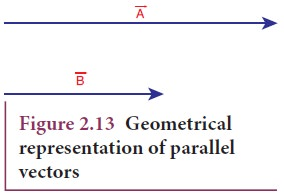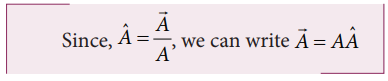Home | | Physics 11th std | Different types of Vectors

# Different types of Vectors

1. Equal vectors 2. Unit vector 3. Orthogonal unit vectors

Different types of Vectors

1. Equal vectors: Two vectorsandare said to be equal when they have equal magnitude and same direction and represent the same physical quantity (Figure 2.12.).a. Collinear vectors: Collinear vectors are those which act along the same line. The angle between them can be 0° or 180°.

i. Parallel Vectors: If two vectorsandact  in the same direction along the same line or on parallel line, then the angle between them is 00 (Figure 2.13).ii. Anti-parallel vectors: Two vectorsandare said to be anti-parallel when they are in opposite directions along the same line or on parallel lines. Then the angle between them is 180o (Figure 2.14).2. Unit vector: A vector divided by its magnitude is a unit vector. The unit vector foris denoted by Aˆ (read as A cap or A hat). It has a magnitude equal to unity or one.Thus, we can say that the unit vector specifies only the direction of the vector quantity.

3. Orthogonal unit vectors: Let iˆ , jˆ and kˆ be three unit vectors which specify the directions along positive x-axis, positive y-axis and positive z-axis respectively. These three unit vectors are directed perpendicular to each other, the angle between any two of them is 90°. iˆ , jˆ and kˆ are examples of orthogonal vectors. Two vectors which are perpendicular to each other are called orthogonal vectors as is shown in the Figure 2.15Study Material, Lecturing Notes, Assignment, Reference, Wiki description explanation, brief detail
11th Physics : UNIT 2 : Kinematics : Different types of Vectors |